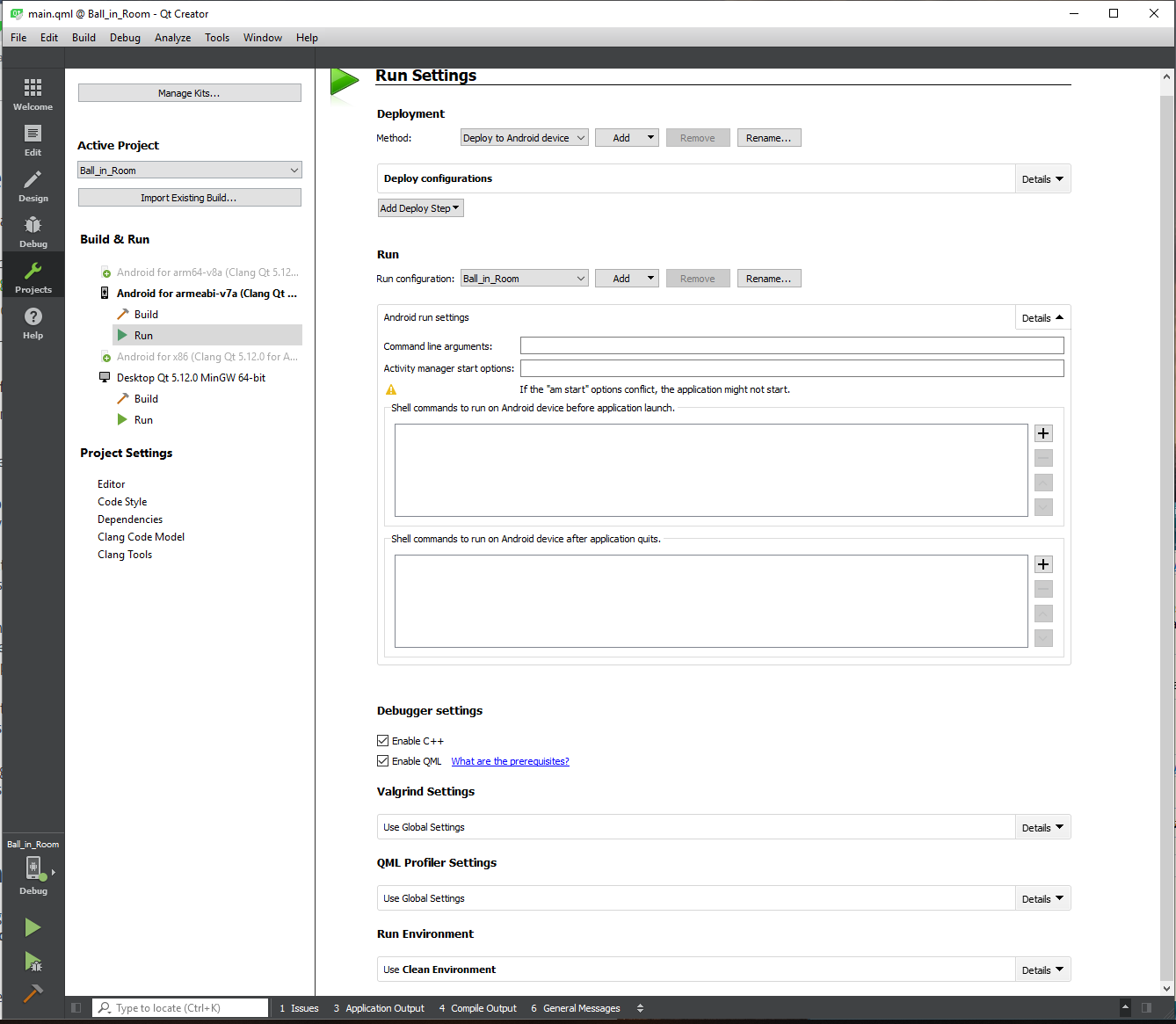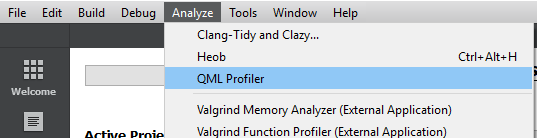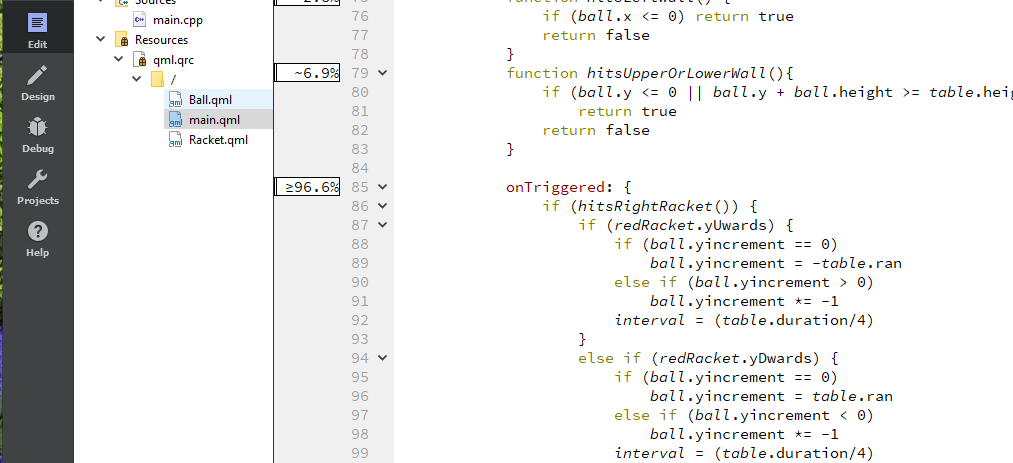# A strange movement effect of a component over another one's speed

• Hi,

Tried well to simply the QML code below to characterise the issue clearly:

`Ball.qml`:

``````import QtQuick 2.12

Rectangle {
width: 18; height: 18
color: "white"

property double xincrement: window.ran
property double yincrement: window.ran
}
``````

`Racket.qml`:

``````import QtQuick 2.12

Rectangle {
id: root
width: 15; height: 65
property int oldY: y
property bool yUwards
property bool yDwards

onYChanged: {
if (y > oldY) yDwards = true
else if (y < oldY) yUwards = true
oldY = y
}

MouseArea {
anchors.fill: root
anchors.margins: -root.height
drag.target: root
drag.axis: Drag.YAxis
drag.minimumY: table.y
drag.maximumY: table.height - root.height - 10
}
}
``````

`main.qml`:

``````import QtQuick 2.12
import QtQuick.Window 2.12
import QtQuick.Controls 2.5

Window {
id: window
visible: true
width: 1200; height: 900
color: "gray"

Rectangle {
id: table
width: window.width / 1.15; height: window.height / 1.15
y: 10
anchors.horizontalCenter: parent.horizontalCenter
property double ran: Math.random() + 0.5
property int duration: 4
property double step: 1.5
color: "royalblue"

Racket {
id: blackRacket
anchors.left: table.left
anchors.leftMargin: width * 2
y: table.height / 2
color: "black"
}
Racket {
id: redRacket
anchors.right: table.right
anchors.rightMargin: width * 2
y: table.height / 2
color: "red"
}
Ball {
id: ball
x: table.width/2
y: table.height/2
}
Timer {
interval: 40; repeat: true; running: true

onTriggered: {
redRacket.yUwards = false
redRacket.yDwards = false
blackRacket.yUwards = false
blackRacket.yDwards = false
}
}
Timer {
interval: table.duration; repeat: true; running: true

function hitsRightRacket() {
if ((ball.x + ball.width >= redRacket.x &&
ball.x <= redRacket.x + redRacket.width) &&
(ball.y + ball.height >= redRacket.y &&
ball.y <= redRacket.y + redRacket.height))
return true
return false
}
function hitsLeftRacket() {
if (((ball.x + ball.width >= blackRacket.x &&
ball.x < blackRacket.x + blackRacket.width)  &&
(ball.y + ball.height >= blackRacket.y &&
ball.y <= blackRacket.y + blackRacket.height))
|| (ball.x <= 0))
return true
return false
}
function hitsRightWall() {
if (ball.x + ball.width >= table.width)
return true
return false
}
function hitsLeftWall() {
if (ball.x <= 0) return true
return false
}
function hitsUpperOrLowerWall(){
if (ball.y <= 0 || ball.y + ball.height >= table.height)
return true
return false
}

onTriggered: {
if (hitsRightRacket()) {
if (redRacket.yUwards) {
if (ball.yincrement == 0)
ball.yincrement = -table.ran
else if (ball.yincrement > 0)
ball.yincrement *= -1
interval = (table.duration/4)
}
else if (redRacket.yDwards) {
if (ball.yincrement == 0)
ball.yincrement = table.ran
else if (ball.yincrement < 0)
ball.yincrement *= -1
interval = (table.duration/4)
}
else {
ball.yincrement = 0
interval = (table.duration/2)
}
ball.xincrement *= -1
}
else if (hitsLeftRacket()) {
if(blackRacket.yUwards) {
if (ball.yincrement == 0)
ball.yincrement = -table.ran
else if (ball.yincrement > 0)
ball.yincrement *= -1
interval = (table.duration/4)
}
else if (blackRacket.yDwards) {
if (ball.yincrement == 0)
ball.yincrement = table.ran
else if (ball.yincrement < 0)
ball.yincrement *= -1
interval = (table.duration/4)
}
else {
ball.yincrement = 0
interval = (table.duration/2)
}
ball.xincrement *= -1
}
else if (hitsRightWall())
ball.xincrement *= -1
else if (hitsLeftWall())
ball.xincrement *= -1
else if (hitsUpperOrLowerWall())
ball.yincrement *= -1

// Move Ball
ball.x = ball.x + (ball.xincrement * table.step);
ball.y = ball.y + (ball.yincrement * table.step);
}
}
}
}
``````

I tested that on my Android device. The problem is that, when rackets are not moving the ball moves smoothly (and it's OK), but when I move either racket, it affects the speed of the ball, strangely! These two must be independent in terms of movement and speed, but in effect this issue takes place. I don't know why.

Will you test this code on your Android device too, to see if the problem exits there as well. If yes, what could be the source of the issue and how to remedy that, please?

Thanks beforehand.

• hi @tomy

hard to tell, I don't think your example will compile, the `table` class seems to be missing.

However, this seems to be the right place to look into QML-Profilling

That will give you a detailed analyzation of what takes how much time, at least on the QML side of the application.

• @J.Hilk

hard to tell, I don't think your example will compile, the table class seems to be missing.

Hi,

But it compiles. And `table` is the id of a Rectangle. Please look at the line 12 of `main.qml`.

Still do I need to study that long paper?

• But it compiles. And `table` is the id of a Rectangle. Please look at the line 12 of `main.qml`.

Still do I need to study that long paper?

Oh, I'm sorry, I did not see that.
Can't test it myself, as I don't have an android device with me these days.

I would recommend studying it, it will go a long way in optimizing your current and any following QML-based project!

• @J.Hilk

Can't test it myself, as I don't have an android device with me these days.

Will you test it on any iOS smartphone which might be to your hands? The Desktop kit handles that well but I'm almost sure it keeps the issue on smartphone operating systems.

I would recommend studying it, it will go a long way in optimizing your current and any following QML-based project!

Thanks. I will begin studying that too.

• In Using QML Profiler I can't find Analyze > QML Profiler to profile the current application!• @tomy
did you check in the menu bar ?• I read the beginning part of that paper and it's to me detailed and useful for advanced devs but doesn't make an impression on the behavior of the program based on the output of starting the application from the QML Profiler. I've saved the trace file and it shows that the program mainly (more than %96) is dealing with the last onTriggered part:I also tried to change the code to some little extent by separating the `table` as a single component, not covering other components in the code anymore. But overall, no changes on the Android device yet!

• I think I found the source of the issue:

The last `Timer` in `main.qml` each time, alongside with moving the ball, needs also to measure the positions of the rackets for collision checks, and while the rackets are moving by the user, those positions (x, y) change, and it makes those snags for the ball's movement.

For that, I tried to define another `Timer` to separate the Ball's movement from collision checks. That is, one `Timer` merely for movement of the Ball, and another for collision checks.
But unfortunately, it couldn't solve the issue either!

As an another attempt, I went for using `property binding` rather than collision checks' `Timer`. I only used a simple `Timer` for moving the Ball, but once again, unfortunately, it couldn't solve the problem. :(

I'm not sure if the problem is with the code or the operating system. Because, the program works fine on the Desktop kit (on Windows) but when tested on my Android version 4.4.2 tablet, the problem comes up.

Here's the new version of the code. I tried to commentate important parts of that so that it reads easily.

If you don't want to read the code, at least test it on your Android/iOS device to see whether it's a code issue or platform problem, please.

`Ball.qml` and `Racket.qml` are as before and here is `main.qml`:

``````import QtQuick 2.12
import QtQuick.Window 2.12
import QtQuick.Controls 2.5

Window {
id: window
visible: true
width: 1000; height: 800
color: "gray"
property double ran: Math.random() + 0.5
property int duration: 4
property double step: 1.5

// The components
// --------------

Rectangle {
id: table
width: window.width / 1.15; height: window.height / 1.15
y: 10
anchors.horizontalCenter: parent.horizontalCenter
color: "royalblue"
}
Racket {
id: blackRacket
anchors.left: table.left
anchors.leftMargin: width * 2
y: table.height / 2
color: "black"
}
Racket {
id: redRacket
anchors.right: table.right
anchors.rightMargin: width * 2
y: table.height / 2
color: "red"
}
Ball {
id: ball
x: table.width/2
y: table.height/2
}

// A number of bool properties to catch the hit between
// the ball and rackets/walls

property bool hitsRightRacket: {
if ((ball.x + ball.width >= redRacket.x &&
ball.x <= redRacket.x + redRacket.width) &&
(ball.y + ball.height >= redRacket.y &&
ball.y <= redRacket.y + redRacket.height))
return true
return false
}
onHitsRightRacketChanged: {
if(hitsRightRacket) {
if (redRacket.yUwards) {
if (ball.yincrement == 0)
ball.yincrement = -ran
else if (ball.yincrement > 0)
ball.yincrement *= -1
timer.interval = duration / 4
}
else if (redRacket.yDwards) {
if (ball.yincrement == 0)
ball.yincrement = ran
else if (ball.yincrement < 0)
ball.yincrement *= -1
timer.interval = duration / 4
}
else {
ball.yincrement = 0
timer.interval = duration / 2
}
ball.xincrement *= -1
}
}

property bool hitsLeftRacket: {
if (((ball.x + ball.width >= blackRacket.x &&
ball.x < blackRacket.x + blackRacket.width)  &&
(ball.y + ball.height >= blackRacket.y &&
ball.y <= blackRacket.y + blackRacket.height))
|| (ball.x <= 0))
return true
return false
}
onHitsLeftRacketChanged: {
if(hitsLeftRacket) {
if(blackRacket.yUwards) {
if (ball.yincrement == 0)
ball.yincrement = -ran
else if (ball.yincrement > 0)
ball.yincrement *= -1
timer.interval = (duration/4)
}
else if (blackRacket.yDwards) {
if (ball.yincrement == 0)
ball.yincrement = ran
else if (ball.yincrement < 0)
ball.yincrement *= -1
timer.interval = duration / 4
}
else {
ball.yincrement = 0
timer.interval = duration / 2
}
ball.xincrement *= -1
}
}

property bool hitsLeftOrRightWall: {
if (ball.x + ball.width >= table.x + table.width ||
ball.x <= table.x)
return true
return false
}
onHitsLeftOrRightWallChanged: {
if(hitsLeftOrRightWall)
ball.xincrement *= -1
}

property bool hitsUpperOrLowerWall: {
if (ball.y <= table.y || ball.y + ball.height >= table.height)
return true
return false
}
onHitsUpperOrLowerWallChanged: {
if(hitsUpperOrLowerWall)
ball.yincrement *= -1
}

// Timers
//--------

Timer {  // this timer repeatedly sets the four booleans to false so that
// the closest racket upwards/downwards movement is caught
interval: 50; repeat: true; running: true

onTriggered: {
redRacket.yUwards = false
redRacket.yDwards = false
blackRacket.yUwards = false
blackRacket.yDwards = false
}
}

Timer {   // This timer's job is merely moving the ball
id: timer
interval: duration; repeat: true; running: true

onTriggered: {
ball.x += ball.xincrement * step
ball.y += ball.yincrement * step
}
}
}
``````

• hi @tomy

good that you can narrow the issue down.

a suggestions, that should make the code a bit faster

bind the collision check to the x movement of the ball (onXChanged)
currently `hitsLeftRacket` depends on `ball.x` and `ball.y` and `blackRacket.y` each time any of those values changes, the whole expression is reevaluated -> 3 times check, when one would be enough.

``````property bool hitsLeftRacket: false //Initial state
property bool hitsRightRacket: false
property bool hitsLeftOrRightWall: false

Ball {
id: ball
x: table.width/2
y: table.height/2

onXChanged: {
var ballXw = ball.x + ball.width;

if (((ballXw  >= blackRacket.x && ball.x < blackRacket.x + blackRacket.width)  &&
(ball.y + ball.height >= blackRacket.y && ball.y <= blackRacket.y + blackRacket.height))
|| (ball.x <= 0))
hitsLeftRacket = true
else
hitsLeftRacket = false

if (( ballXw  >= redRacket.x && ball.x <= redRacket.x + redRacket.width) &&
(ball.y + ball.height >= redRacket.y && ball.y <= redRacket.y + redRacket.height))
hitsRightRacket= true
else
hitsRightRacket= false

if (ballXw   >= table.x + table.width ||
ball.x <= table.x)
hitsLeftOrRightWall = true
else
hitsLeftOrRightWall = false
}
}
``````

also if you make the Rackets and the ball part of the table (children) than x and y will be relative to the coordinates of the table, would simplify the left or right wall check:

``````hitsLeftOrRightWall  = (ballXw   >= table.width ||  ball.x <=0)
``````

• @J.Hilk
Hi,
Binding the collision check to the Ball's X movement is nicer and more reasonable. I used this.

Making the table the parent of Ball and Rackets won't create that change in the appearance of code. Will it?

``````hitsLeftOrRightWall  = (ballXw  >= table.width ||  ball.x <=0)
// table is parent
hitsLeftOrRightWall  = (ballXw >= table.x + table.width || ball.x <= table.x)
// table isn't parent
``````

Anyway, your suggestion is used in the code as below.
Unfortunately, when the ball hits the lower wall, it doesn't return upwards in practice and the condition for that in the onXChanged's body,
becomes true once again while it shouldn't!

But worse than this, although the code this way doesn't meat the specs of the game, when run on my Android device, the problem with the strange effect of rackets movements over ball's speed still exists! :( :(

It's really odd, and I checked the specs of the component Racket but nothing is clear to make the issue!

``````Window {
id: window
visible: true
width: 1000; height: 800
color: "gray"
property double ran: Math.random() + 0.5
property int duration: 4
property double step: 1.5
property bool hitsLeftRacket: false  //Initial state
property bool hitsRightRacket: false
property bool hitsLeftOrRightWall: false
property bool hitsUpperOrLowerWall: false
property int ballXw: ball.x + ball.width

// The components
// --------------

Rectangle {
id: table
width: window.width / 1.15; height: window.height / 1.15
y: 10
anchors.horizontalCenter: parent.horizontalCenter
color: "royalblue"
}
Racket {
id: blackRacket
anchors.left: table.left
anchors.leftMargin: width * 2
y: table.height / 2
color: "black"
}
Racket {
id: redRacket
anchors.right: table.right
anchors.rightMargin: width * 2
y: table.height / 2
color: "red"
}
Ball {
id: ball
x: table.width/2
y: table.height/2

onXChanged: {
hitsLeftRacket = (((ballXw >= blackRacket.x &&
ball.x < blackRacket.x + blackRacket.width) &&
(ball.y + ball.height >= blackRacket.y &&
ball.y <= blackRacket.y + blackRacket.height))|| (ball.x <= 0))

hitsRightRacket = ((ballXw >= redRacket.x &&
ball.x <= redRacket.x + redRacket.width) &&
(ball.y + ball.height >= redRacket.y &&
ball.y <= redRacket.y + redRacket.height))

hitsLeftOrRightWall = (ballXw >= table.x + table.width ||
ball.x <= table.x)

hitsUpperOrLowerWall = (ball.y <= table.y ||
(ball.y + ball.height >= table.y + table.height))
}
}

Timer {
interval: duration; repeat: true; running: true

onTriggered: {
if(hitsRightRacket) {
if (redRacket.yUwards) {
if (ball.yincrement == 0)
ball.yincrement = -ran
else if (ball.yincrement > 0)
ball.yincrement *= -1
interval = duration / 4
}
else if (redRacket.yDwards) {
if (ball.yincrement == 0)
ball.yincrement = ran
else if (ball.yincrement < 0)
ball.yincrement *= -1
interval = duration / 4
}
else {
ball.yincrement = 0
interval = duration / 2
}
ball.xincrement *= -1
}

else if(hitsLeftRacket) {
if(blackRacket.yUwards) {
if (ball.yincrement == 0)
ball.yincrement = -ran
else if (ball.yincrement > 0)
ball.yincrement *= -1
interval = (duration/4)
}
else if (blackRacket.yDwards) {
if (ball.yincrement == 0)
ball.yincrement = ran
else if (ball.yincrement < 0)
ball.yincrement *= -1
interval = duration / 4
}
else {
ball.yincrement = 0
interval = duration / 2
}
ball.xincrement *= -1
}

else if(hitsLeftOrRightWall) ball.xincrement *= -1

else if(hitsUpperOrLowerWall) ball.yincrement *= -1

ball.x += ball.xincrement * step
ball.y += ball.yincrement * step
}
}

Timer {  // this timer repeatedly sets the four booleans to false so that
// the closest racket upwards/downwards movement is caught
interval: 50; repeat: true; running: true

onTriggered: {
redRacket.yUwards = false
redRacket.yDwards = false
blackRacket.yUwards = false
blackRacket.yDwards = false
}
}
}

``````